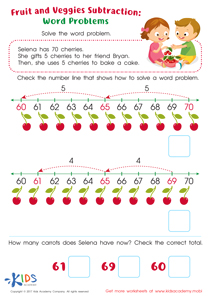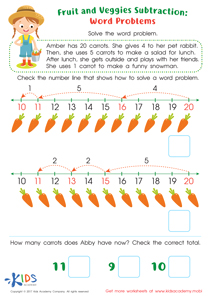# Math Lesson - 2 Step Subtraction Word Problems Using Drawings, Grade 2

• ### Activity 1 / Subtraction Word Problems Free PrintableCheck out this subtractions words problems free printable worksheet that offers your child a different strategy to use while solving challenging subtraction word problems.
After reading the word problem, your child will use number lines as a strategy to solve the word problem with ease!

• ### Activity 2 / Subtraction Word Problems PrintableSubtraction word problems for 2nd grade help kids use subtraction to solve problems in a different way to boost their sense of numeracy.
This fun fruit and veggies subtraction worksheet features number lines to give your child yet another technique to solve tricky subtraction word problems!

• ### Activity 3 / Step Subtraction Word Problems WorksheetWhen it comes to word problems, one of the most important strategies is to be able to see the problem in your head. This adorable PDF worksheet will let your child have concrete imagery to help solve this multiple-step subtraction problem. Using one-to-one number representation, they'll help Gina figure out how many rockets she has left, and they'll be successful problem solvers too!